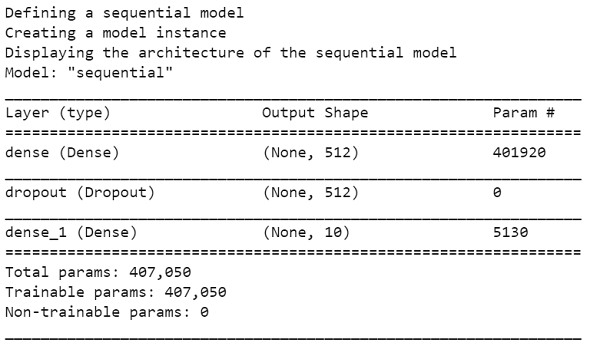# How can Tensorflow be used to define a model for MNIST dataset?

Tensorflow is a machine learning framework that is provided by Google. It is an open−source framework used in conjunction with Python to implement algorithms, deep learning applications and much more. It has optimization techniques that help in performing complicated mathematical operations quickly. This is because it uses NumPy and multi−dimensional arrays. These multi−dimensional arrays are also known as ‘tensors’. The framework supports working with deep neural network.

The ‘tensorflow’ package can be installed on Windows using the below line of code −

pip install tensorflow

Tensor is a data structure used in TensorFlow. It helps connect edges in a flow diagram. This flow diagram is known as the ‘Data flow graph’. Tensors are nothing but multidimensional array or a list.

Keras is a deep learning API, which is written in Python. It is a high-level API that has a productive interface that helps solve machine learning problems. It runs on top of Tensorflow framework. It was built to help experiment in a quick manner. Keras is already present within the Tensorflow package. It can be accessed using the below line of code.

import tensorflow
from tensorflow import keras

We are using the Google Colaboratory to run the below code. Google Colab or Colaboratory helps run Python code over the browser and requires zero configuration and free access to GPUs (Graphical Processing Units). Colaboratory has been built on top of Jupyter Notebook. Following is the code snippet −

## Example

print("Defining a sequential model")
def create_model():
model = tf.keras.models.Sequential([
keras.layers.Dense(512, activation='relu', input_shape=(784,)),
keras.layers.Dropout(0.2),
keras.layers.Dense(10)
])

loss=tf.losses.SparseCategoricalCrossentropy(from_logits=True),
metrics=[tf.metrics.SparseCategoricalAccuracy()])

return model

print("Creating a model instance")
model = create_model()

print("Displaying the architecture of the sequential model")
model.summary()

## Output## Explanation

• A sequential model is created using Keras.

• The layers are created which are ‘dense’.

• This model is compiled.

• An instance of this model is created.